Section 3 1 Scatterplots TwoVariable Quantitative Data Most

• Slides: 16Section 3. 1 Scatterplots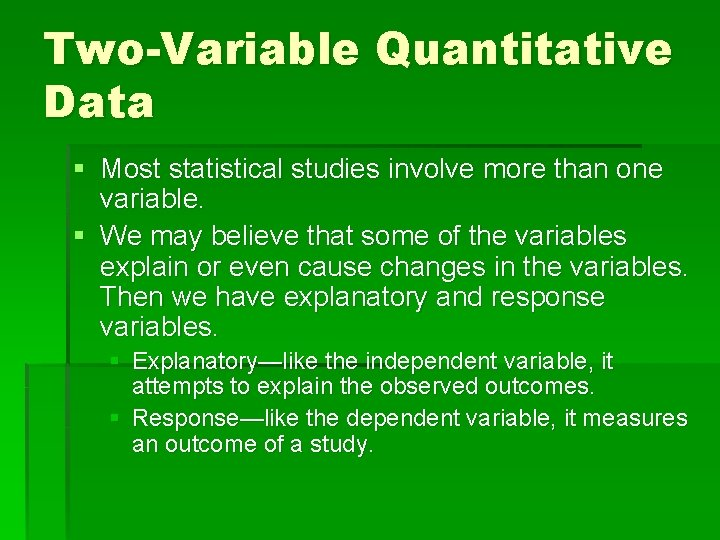Two-Variable Quantitative Data § Most statistical studies involve more than one variable. § We may believe that some of the variables explain or even cause changes in the variables. Then we have explanatory and response variables. § Explanatory—like the independent variable, it attempts to explain the observed outcomes. § Response—like the dependent variable, it measures an outcome of a study.Examples Identify the explanatory and response variables: • Alcohol causes a drop in body temperature. To measure this, researchers give several different amounts of alcohol to mice, then measure the change in their body temperature after 15 minutes. • If an object is dropped from a height, then its downward speed theoretically increases over time due to the pull of gravity. To test this, a ball is dropped and at certain intervals of time, the speed of the ball is measured.Scatterplots § § Used for two-variable quantitative data! Explanatory variable goes on the x-axis Response variable goes on the y-axis The explanatory variable does not necessarily “CAUSE” the change in the response variable.§ D i s p l a y i n g R e l a ti o n s h i p s : S c a tte r p l o ts Since Body weight is our e. Xplanatory v a r i a b l e , b e s u r e t o p l a c e i t o n t h e X a x i s ! Body weight (lb) 120 187 109 103 131 165 158 116 Backpack weight (lb) 26 30 26 24 29 35 31 28 S c a t t e r p lo t s a n d C o r r e la t io n Make a scatterplot of the relationship between body weight a n d p a ck w e i g h t.Interpreting Graphs One Variable Quantitative Data Center Two-Variable Quantitative Data Form Linear? Clusters? Gaps? Shape Direction Positive? Negative? Spread Strength Strong? Weak? Moderate? OutliersIn sentence form… § There is a (strong/weak), (positive/negative), (linear/non-linear) relationship between (your two variables).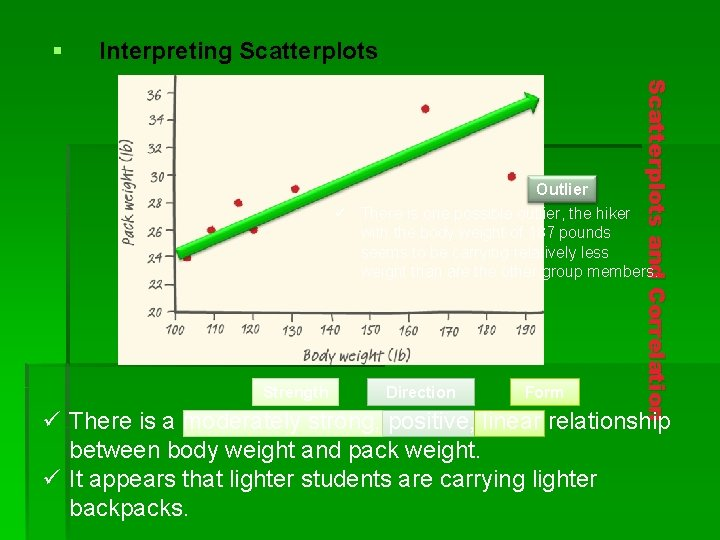§ I n te r p r e ti n g S c a tte r p l o ts S c a t t e r p lo t s a n d C o r r e la t io n Outlier ü There is one possible outlier, the hiker with the body weight of 187 pounds seems to be carrying relatively less weight than are the other group members. Strength Direction Form ü There is a moderately strong, positive, linear relationship between body weight and pack weight. ü It appears that lighter students are carrying lighter backpacks.Adding Categorical Variables to Scatterplots § You can use different plotting symbols or different colors to designate a categorical variable. § You still have two quantitative variables, but you can add a “category” to these variables.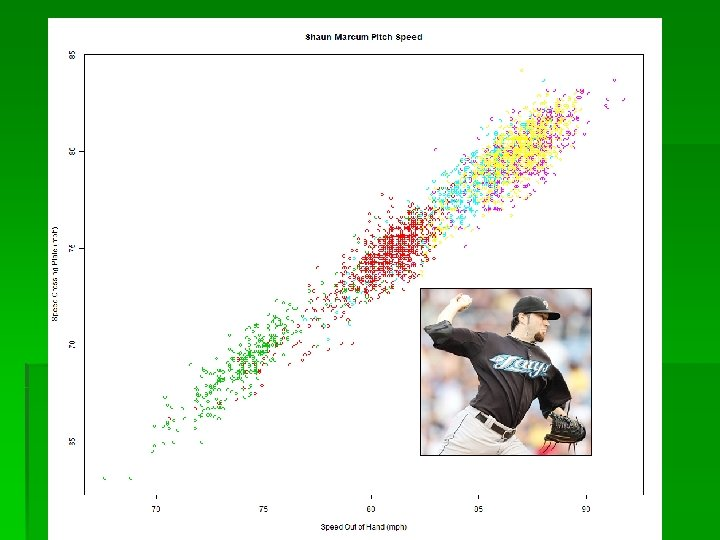Some quick tips for drawing scatterplots § Choose an appropriate scale for the axes. Use a break if appropriate. § Label, Label… § If you are given a grid, try to use a scale that will make the scatterplot use the whole grid.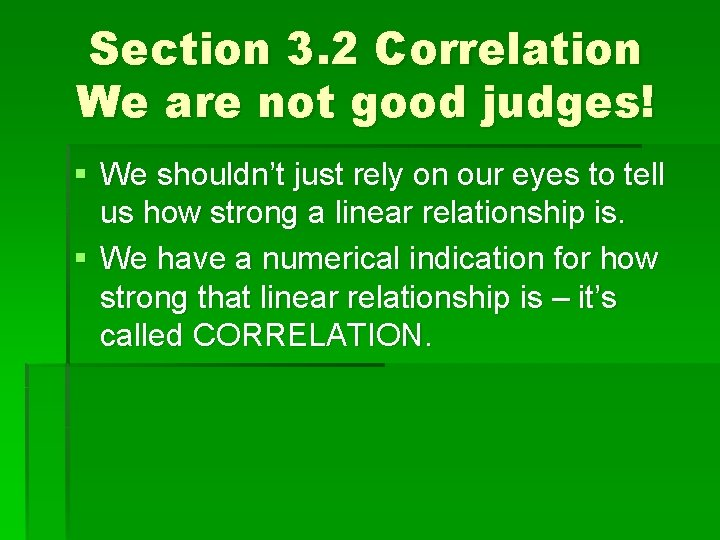Section 3. 2 Correlation We are not good judges! § We shouldn’t just rely on our eyes to tell us how strong a linear relationship is. § We have a numerical indication for how strong that linear relationship is – it’s called CORRELATION.Definition: The correlation r measures the strength of the linear relationship between two quantitative variables. • r is always a number between -1 and 1 • r > 0 indicates a positive association. • r < 0 indicates a negative association. • Values of r near 0 indicate a very weak linear relationship. • The strength of the linear relationship increases as r moves away from 0 towards -1 or 1. • The extreme values r = -1 and r = 1 occur only in the case of a perfect linear relationship. S c a t t e r p lo t s a n d C o r r e la t io nFacts About Correlation § It does not require a response and explanatory variable. Ex. How are SAT math and verbal scores related? § If you switch the x and the y variables, the correlation doesn’t change. § If you change the units of measurement for x and/or y, the correlation doesn’t change. § Positive r values indicate a positive relationship; negative values indicate a negative relationship. Remember… not cause.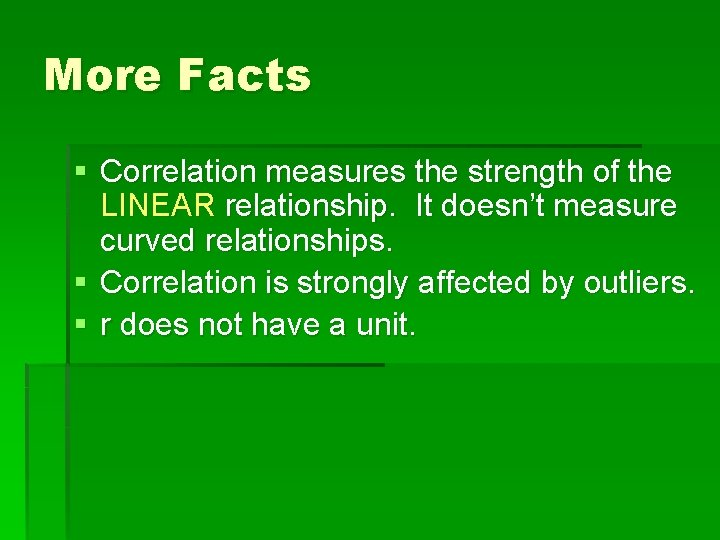More Facts § Correlation measures the strength of the LINEAR relationship. It doesn’t measure curved relationships. § Correlation is strongly affected by outliers. § r does not have a unit.Homework Chapter 3 #11, 13, 14, 15, 17, 20, 22, 26